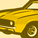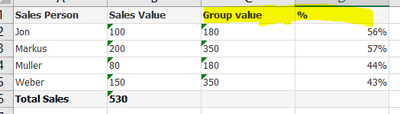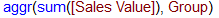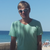# QlikView App Dev

Discussion Board for collaboration related to QlikView App Development.

Announcements
Join us at the Cloud Data and Analytics Tour! REGISTER TODAY
cancel
Showing results for
Search instead for
Did you mean:Creator

## AGGR in Chart

Hi, I have a table like thisI want to have report like thisbut i am not able to get the proper result in QLikView report.

Qlikview reporti am using following expressions for Group value =if i write the expressions as Calculated dimension. that works fine. but with that i can not get  %. I can do group by in Load script but i want to do it in Chart. Is it possible? Any suggestion will be highly appreciated.

Thank

Kazi

Labels (3)

• ### Scripting

1 Solution

Accepted SolutionsMaster II

Dimension:

``Sales Person``

Exp for "Group value as Expression"

``sum(aggr(sum(Total <Group> [Sales Value]), Group,[Sales Person]))``

Exp for "%":

``sum([Sales Value]) / sum(aggr(sum(Total <Group> [Sales Value]), Group,[Sales Person]))  ``
1 ReplyMaster II

Dimension:

``Sales Person``

Exp for "Group value as Expression"

``sum(aggr(sum(Total <Group> [Sales Value]), Group,[Sales Person]))``

Exp for "%":

``sum([Sales Value]) / sum(aggr(sum(Total <Group> [Sales Value]), Group,[Sales Person]))  ``